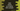# Java Example to empty an Arraylist## Empty an arraylist in Java using clear() and removeAll() :

To remove all elements from an ArrayList in Java, we have two inbuilt methods : clear() and removeAll(). Both methods can remove all elements from an ArrayList. Both method looks same but there is a difference between them. Even both of them performs differently.

In this tutorial, we will show you how to empty an ArrayList using clear and removeAll method and later, we will discuss about their differences.

### Using clear() :

First of all, let’s try to clear one arraylist using clear() method. It will look like as below :

``````import java.util.ArrayList;

public class Main {
public static void main(String args[]) {
//1
ArrayList myList = new ArrayList<>();

//2
for (int i = 0; i < 10; i++) {
}

//3
System.out.println("ArrayList : " + myList);

//4
myList.clear();
System.out.println("Final ArrayList : " + myList);
}
}``````

### Explanation :

The commented numbers in the above example denote the step number below :

1. Create one ArrayList myList that can store only string values.
2. Run one for loop starting from i = 0 to i = 10. For each iteration, add the string representation of the value of i to the arraylist.
3. Print out the value of myList.
4. Clear the ArrayList and print out its values again.

It will print one output like below :

``````ArrayList : [0, 1, 2, 3, 4, 5, 6, 7, 8, 9]
Final ArrayList : []``````

### Using removeAll() :

Now, let’s try to remove all elements from an ArrayList using removeAll() method. It looks like as below :

``````import java.util.ArrayList;

public class Example {
public static void main(String args[]) {
ArrayList myList = new ArrayList<>();

for (int i = 0; i < 10; i++) {
}

System.out.println("ArrayList : " + myList);

myList.removeAll(myList);
System.out.println("Final ArrayList : " + myList);
}
}``````

It will print the same output as the above program. removeAll() takes one Collection parameter and removes all the elements that contains in the parameter is removed from the collection where we are calling this method. So, if both of these elements are the same list, it will remove all elements from the list.

### Comparing performance between clear() and removeAll() :

To compare the performance between these two methods, let’s take a look at the source code of these functions : clear() :

``````public void clear() {
for (int i = 0; i < size; i++)
arraylist[i] = null;

size = 0;
}``````

removeAll() :

``````public boolean removeAll(Collection c) {
boolean ismodified = false;
Iterator iterator = iterator();
while (iterator.hasNext()) {
if (c.contains(iterator.next())) {
iterator.remove();
ismodified = true;
}
}
return ismodified;
}``````

As you can see that the first method is of O(n) complexity and the second one is of O(n^2). So, for better performance, we can use clear() instead of removeAll().

### Conclusion :

In this tutorial, we have learn how to empty an arraylist in Java using clear() and removeAll() functions. Also, we have seen that clear() is better in terms of permance than removeAll. Try to run the above exmaples and drop a comment below if you have any queries.